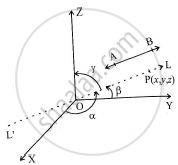HSC Arts 12th Board ExamMaharashtra State Board
Share

# If l, m, n are the direction cosines of a line, then prove that l2 + m2 + n2 = 1. Hence find the direction angle of the line with the X axis which makes direction angles of 135° and 45° with Y and Z axes respectively. - HSC Arts 12th Board Exam - Mathematics and Statistics

ConceptDirection Cosines and Direction Ratios of a Line

#### Question

If l, m, n are the direction cosines of a line, then prove that l2 + m2 + n2 = 1. Hence find the
direction angle of the line with the X axis which makes direction angles of 135° and 45° with Y and Z axes respectively.

#### Solution

Let alpha,beta,gamma  be the angles made by the line with X-, Y-, Z- axes respectively.l=cosalpha, m=cosbeta and n=cosgamma

Let bara=a_1hati+a_2hatj+a_3hatk be any non-zero vector along the line.

Since hati is the unit vector along X-axis,

bara.hati=|bara|.|hati|cosalpha=acosalpha

Also, bara.hati=(a_1hati+a_2hatj+a_3hatk).hati

=a_1xx1+a_2xx0+a_3xx0=a_1

acosalpha=a_1 ..............................(1)

Since hatj is the unit vector along Y-axis,

bara.hatj=|bara|.|hatj|cosbeta=acosbeta

bara.hatj=(a_1hati+a_2hatj+a_3hatk).hatj

=a_1xx0+a_2xx1+a_3xx0=a_2

acosbeta=a_2 ......................(2)

similarly acosgamma=a_3 .............(3)

from equations (1), (2) and (3),

a^2cos^2alpha+a^2cos^2beta+a^2cos^2gamma=a_1^2+a_2^2+a_3^2

a^2(cos^2alpha+cos^2beta+cos^2gamma)=a^2                [a=|bara|=sqrt(a_1^2+a_2^2+a_3^2)]

therefore cos^2alpha+cos^2beta+cos^2gamma=1

i.e l^2+m^2+n^2=1

also

alpha=?, beta=135^@,gamma=45^@

cos^2alpha+cos^2beta+cos^2gamma=45^@

cos^2alpha+cos^2 135^@+cos^2 45^@=1

cos^2alpha+1/2+1/2=1

cos^alpha=0

therefore alpha=pi/2 or (3pi)/2

Is there an error in this question or solution?

#### APPEARS IN

2016-2017 (March) (with solutions)
Question 3.2.1 | 4.00 marks
Solution If l, m, n are the direction cosines of a line, then prove that l2 + m2 + n2 = 1. Hence find the direction angle of the line with the X axis which makes direction angles of 135° and 45° with Y and Z axes respectively. Concept: Direction Cosines and Direction Ratios of a Line.
S